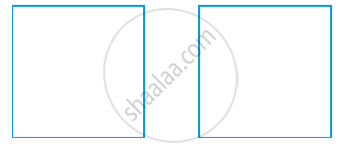Share

# Congruence of Triangles

#### notes

'A triangle has three sides , three angles & three vertices.' 'tri' means three .
‘congruent’ means equal in all respects or figures whose shapes and sizes are both the same.
For instance , one square on the other with sides of the same measure in following fig.or by placing two equilateral triangles of equal sides on each other. You will observe that the squares are congruent to each other and so are the equilateral triangle.
two triangles are congruent if the sides and angles of one triangle The are equal to the corresponding sides and angles of the other triangle.
Note that in congruent triangles corresponding parts are equal and we write in short ‘CPCT’ for corresponding parts of congruent triangles.

#### description

• Properties of Congruent Triangle
1.   ASA Test
2.   SAS Test
3. SSS Test
4. Hypotenuse Side Test
• Corresponding angles of congruent triangles

### Shaalaa.com

Congruence of Triangles [00:09:39]
S
0%

S